# Gauss' law (magnetism)

In physics, more specifically in electromagnetism, Gauss' magnetic law states that magnetic monopoles (magnetic charges) do not exist. More technically, Gauss' law states that the integral vanishes of the magnetic induction B over any closed surface. Expressed in the form of an equation Gauss' law for magnetism reads: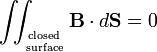$\iint_{\mathrm{closed}\atop\mathrm{surface}} \mathbf{B}\cdot d \mathbf{S} = 0$

Here dS is an vector with length dS, the area of an infinitesimal surface element on the closed surface, and direction perpendicular to the surface element dS, pointing outward. The vector B is the magnetic induction (in vacuum proportional to the magnetic field H) at the position dS, the dot indicates a dot product between the vectors B and dS. The double integral is over a closed surface that may envelop one or more permanent magnets and electric current carrying wires. The law is called after the German mathematician Carl Friedrich Gauss.

By application of the divergence theorem Gauss' law becomes one of Maxwell's equations, namely, the divergence of the field B is zero everywhere,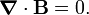$\boldsymbol{\nabla}\cdot \mathbf{B} = 0.$

## Magnetic monopole

A surface integral of B is often called magnetic flux denoted by Φ,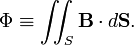$\Phi \equiv \iint_{S} \mathbf{B}\cdot d \mathbf{S}.$

In electrostatics one defines an electric flux Ψ,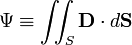$\Psi \equiv \iint_{S} \mathbf{D}\cdot d \mathbf{S}$

where the displacement D is equal to the electric field E times ε. The latter quantity is the relative permittivity εr times the electric constant ε0, ε = ε0εr. Gauss' law for electrostatics states that electric charge is the source of flux through a closed surface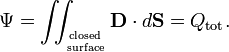$\Psi = \iint_{\mathrm{closed}\atop\mathrm{surface}} \mathbf{D}\cdot d \mathbf{S} = Q_\mathrm{tot}.$

Since the corresponding integral of B over a closed surface is always zero, the magnetic version of the Gauss law states that a magnetic charge (magnetic monopole) does not exist, neither as permanent monopole, nor as monopole induced by an electric current. This law expresses the empirical fact that division of a permanent magnet (a magnetic dipole) into two pieces gives again two magnetic dipoles, and not a separate North pole and South pole (the equivalence of a plus and minus electric charge). It was Coulomb who first investigated this systematically and came to the conclusion that a magnetic charge does not exist.